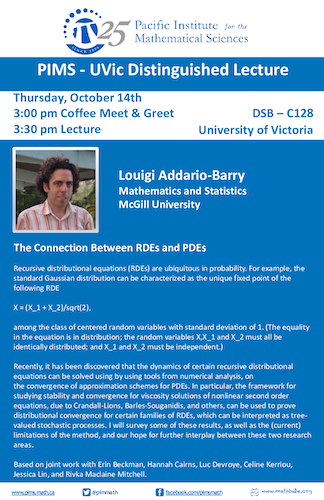PIMS - UVictoria Distinguished Colloquium: Louigi Addario-Berry

• Date: 10/14/2021
Speaker(s):
Location:

TBA

Topic:

The Connection Between RDEs and PDEs

Description:

Recursive distributional equations (RDEs) are ubiquitous in probability. For example, the standard Gaussian distribution can be characterized as the unique fixed point of the following RDE
$$X = (X_1 + X_2)/\sqrt(2),$$
among the class of centered random variables with standard deviation of 1. (The equality in the equation is in distribution; the random variables $$X ,X_1$$ and $$X_2$$ must all be identically distributed; and $$X_1$$ and $$X_2$$ must be independent.)

Recently, it has been discovered that the dynamics of certain recursive distributional equations can be solved using by using tools from numerical analysis, on the convergence of approximation schemes for PDEs. In particular, the framework for studying stability and convergence for viscosity solutions of nonlinear second order equations, due to Crandall-Lions, Barles-Souganidis, and others, can be used to prove distributional convergence for certain families of RDEs, which can be interpreted as tree- valued stochastic processes. I will survey some of these results, as well as the (current) limitations of the method, and our hope for further interplay between these two research areas.Based on joint work with Erin Beckman, Hannah Cairns, Luc Devroye, Celine Kerriou, Jessica Lin, and Rivka Maclaine Mitchell.

Other Information:
• Date: Thursday, October 14th
• 3:00 pm Coffee Meet & Greet
• 3:30 pm Lecture
• Location: DSB - C128